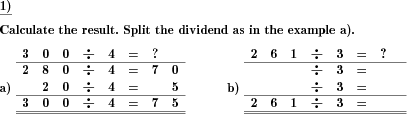Custom math worksheets at your fingertips# Details for problem "Division, perform by splitting dividend"

Quickname: 6543

Elementary School, Primary School.

## Summary

Divide by splitting the dividend.

## Example## Description

Dividing positive whole numbers can be trained using a splitting technique.

The dividend, which has two or three places, has to be split into the summands, one of which is a multiple of ten where the divisor is a multiple of ten, too. The divisor is always in the range from two to nine. The divisor is split into two summands, one which is a multiple of ten and another, which is the ones position.

The problem can be configured to provide the summands the divided is split into. A sample problem with the answer included can be included to illustrate the expected answer.

The number of problems can be selected.

Download free printable worksheets for this math problem here. The worksheet contains the problems only, the solution sheet includes the answers. Just click on the respective link.

•Worksheet 1Solution sheet with answers
•Worksheet 2Solution sheet with answers
•Worksheet 3Solution sheet with answers

If you can not see the solution sheets for download, they may be filtered out by an ad blocker that you may have installed. If this is the case, please allow ads for this page and reload the page. The solution sheets will then reappear.

• Do these sample worksheets do not really fit?
• Do you need more math worksheets, with a different level of difficulty?
• Would you like to combine different problems on a worksheet and adjust them to your needs?
• As a teacher, you can put together your own worksheets using the automatically generated math problems provided.
With a free initial credit, you can start creating your own math worksheets in a few minutes.

You can try it for free! Register here, to create custom worksheets now!

## Customization options for this problem

Parameter
Possible values
Number of problems
1, 2, 3, 4, 5, 6, 7, 8, 9, 10
Divisor
all, 2, 3, 4, 5, 6, 7, 8, 9
Split dividends
show, hide one, hide both, random
Sample problem
Yes, No

## Similar problems

Remark
Description
As a generic division problem
Division of two whole numbers, with remainder, written long division method illustrated.
Also available in the form of a multiplication task
Preparation for long multiplication: Multiply by decomposing first factorDeutsche Version dieser Aufgabe
These informational pages with samples describe math problems that can be combined on custom math worksheets with solutions for home and K-12 school use.
Deutsche Seiten
×